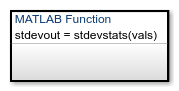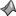## Reuse MATLAB Code by Defining MATLAB Functions

A MATLAB® function in a Stateflow® chart is a graphical element that you use to write algorithms that are easier to implement by calling built-in MATLAB functions. Typical applications include:

• Matrix-oriented calculations

• Data analysis and visualization

This type of function is useful for coding algorithms that are more easily expressed by using MATLAB instead of the graphical Stateflow constructs. MATLAB functions also provide optimizations for generating efficient, production-quality C code for embedded applications.

A MATLAB function can reside anywhere in a chart, state, or subchart. The location of the function determines the set of states and transitions that can call the function.

• If you want to call the function within one state or subchart and its substates, put your MATLAB function in that state or subchart. That function overrides any other functions of the same name in the parents and ancestors of that state or subchart.

• If you want to call the function anywhere in a chart, put your MATLAB function at the chart level.

• If you want to call the function from any chart in your model, put your MATLAB function at the chart level and enable exporting of chart-level functions. For more information, see Export Stateflow Functions for Reuse.

Note

A MATLAB function can access chart and state data above it in the Stateflow hierarchy.

For example, this MATLAB function has the name `stdevstats`. It takes an argument `vals` and returns an output value `stdevout`.To compute the standard deviation of the values in `vals`, the function uses this code.

```function stdevout = stdevstats(vals) %#codegen % Calculates the standard deviation for vals len = length(vals); stdevout = sqrt(sum(((vals-avg(vals,len)).^2))/len); function mean = avg(array,size) mean = sum(array)/size;```

### Define a MATLAB Function in a Chart

1. In the object palette, click the MATLAB function icon.

2. On the chart canvas, click the location for the new MATLAB function.

3. Enter the signature label for the function.

The signature label of the function specifies a name for your function and the formal names for its arguments and return values. A signature label has this syntax:

``[return_val1,return_val2,...] = function_name(arg1,arg2,...)``
You can specify multiple return values and multiple input arguments. Each return value and input argument can be a scalar, vector, or matrix of values. For functions with only one return value, omit the brackets in the signature label.

You can use the same variable name for both arguments and return values. When you use the same variable for an input and output, you create in-place data. For example, a function with this signature label uses the variables `y1` and `y2` as both inputs and outputs:

`[y1,y2,y3] = f(y1,u,y2)`
If you export this function to C code, the generated code treats `y1` and `y2` as in-place arguments passed by reference. Using in-place data reduces the number of times that the generated code copies intermediate data, which results in more efficient code.

In the Symbols pane and the Model Explorer, the arguments and return values of the function signature appear as data items that belong to your function. Arguments have the scope `Input`. Return values have the scope `Output`.

4. Specify the data properties for each argument and return value, as described in Set Data Properties. When an argument and a return value have the same name, you can edit properties only for the argument. The properties for the return value are read-only.

5. To program the function, open the MATLAB Function Block Editor by double-clicking the function box.

6. In the MATLAB Function Block Editor, enter the MATLAB code implementing your function. For more information, see Program a MATLAB Function in a Chart.

Your function can access its own data or data belonging to parent states or the chart. The data items in the function can have one of these scopes:

• `Constant` — Constant data retains its initial value through all function calls.

• `Parameter` — Parameter data retains its initial value through all function calls.

In MATLAB functions, you do not need to create local or temporary function data explicitly. Instead, you can use undefined variables to store values that are accessible only during the rest of the function call. To store values that persist across function calls, use local data at the chart level or use the keyword `persistent`.

### Call MATLAB Functions in States and Transitions

You can call MATLAB functions from the actions of any state or transition or from other functions. If you export a MATLAB function, you can call it from any chart in the model. For more information about exporting functions, see Export Stateflow Functions for Reuse.

To call a MATLAB function, use the function signature and include an actual argument value for each formal argument in the function signature.

``[return_val1,return_val2,...] = function_name(arg1,arg2,...)``

If the data types of the actual and formal arguments differ, the function casts the actual argument to the type of the formal argument.

### Specify Properties of MATLAB Functions

The properties listed below specify how a MATLAB function interacts with the other components in your Stateflow chart. You can modify these properties in the Property Inspector, the Model Explorer, or the MATLAB Function properties dialog box.

To use the Property Inspector:

1. In the Modeling tab, under Design Data, select .

2. In the Stateflow Editor, select the MATLAB function.

3. In the Property Inspector, edit the transition properties.

To use the Model Explorer:

1. In the Modeling tab, under Design Data, select .

2. In the Model Hierarchy pane, select the MATLAB function.

3. In the Dialog pane, edit the MATLAB function properties.

To use the MATLAB Function properties dialog box:

1. In the Stateflow Editor, right-click the MATLAB function.

2. Select Properties.

3. In the properties dialog box, edit the MATLAB function properties.

You can also modify these properties programmatically by using `Stateflow.EMFunction` objects. For more information about the Stateflow programmatic interface, see Overview of the Stateflow API.

#### Name

Function name. Click the function name link to open your function in the MATLAB Function Block Editor.

#### Function Inline Option

Controls the inlining of your function in generated code:

• `Auto` — Determines whether to inline your function based on an internal calculation.

• `Inline` — Inlines your function if you do not export it to other charts and it is not part of a recursion. (A recursion exists if your function calls itself directly or indirectly through another function call.)

• `Function` — Does not inline your function.

This property is not available in the Property Inspector.

#### Label

Signature label for your function. The function signature label specifies a name for your function and the formal names for its arguments and return values. This property is not available in the Property Inspector.

#### Saturate on Integer Overflow

Specifies whether integer overflows saturate in the generated code. For more information, see Handle Integer Overflow for Chart Data.

This property applies only to MATLAB functions in charts that use C as the action language. In charts that use MATLAB as the action language, the behavior of data depends on the value of the Saturate on integer overflow property for the chart.

This property is not available in the Property Inspector.

#### MATLAB Function `fimath`

Defines the `fimath` properties for the MATLAB function. The `fimath` properties specified are associated with all `fi` and `fimath` objects constructed in the MATLAB function. Choose one of these options:

• Same as MATLAB — The function uses the same `fimath` properties as the current global `fimath`. The edit box appears dimmed and displays the current global `fimath` in read-only form. For more information on the global `fimath` and `fimath` objects, see the Fixed-Point Designer™ documentation.

• Specify Other — Specify your own `fimath` object by one of these methods:

• Construct the `fimath` object inside the edit box.

• Construct the `fimath` object in the MATLAB or model workspace and enter its variable name in the edit box.

This property applies only to MATLAB functions in charts that use C as the action language. In charts that use MATLAB as the action language, the behavior of data depends on the value of the MATLAB Chart fimath property for the chart.

This property is not available in the Property Inspector.

#### Description

Description of the MATLAB function.